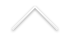Data: 24/05/2017
Hora: 13:30h
Local: C119

Palestrante: Dimas Percy Abanto Silva (IMPA)

Título: Some results of conformally invariant equations

Resumo: This work is about conformally invariant equations from a geometric point of view. In other words, given a solution to an elliptic conformally invariant equation in a subdomain of the sphere, following ideas of Espinar-Galvez-Mira, we construct an elliptic hypersurface in the Hyperbolic space. We can related analytic conditions of the solution to the conformally invariant elliptic equation and the geometry of the hypersurface. In this work, we show some results for degenerate elliptic problems for
conformal metrics in dimension n > 2. More precisely, we show a non- existence theorem for degenerate elliptic problems for conformal metrics on the closed northern hemisphere of the n-sphere with minimal boundary. On the compact annulus in the n-sphere, we prove a uniqueness result for degenerate problem with minimal boundary. Also, we prove a non-existence theorem for degenerate problems on the compact annulus in the n-sphere under the hypothesis that there is a solution on the closed northern hemisphere of the n-sphere minus the north pole such that satis es certain property. This is a work with Jose Espinar.